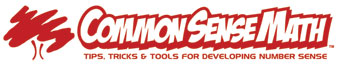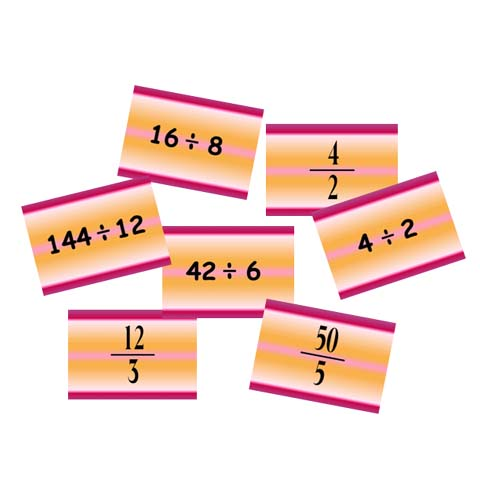STANDARD:  Number Sense  LEVELS: E  EM  M   PURPOSE:  To determine which basic division facts students know - not by using crutches, but actually know in an appropriate amount of time requiring a quick accurate knowledge of basic facts.   STRATEGY:Understand that division can be written in Vertical and Horizontal form, as well as under the "box" like elementary school.   DIRECTIONS:Write the correct answer before the slide changes.  IF you don't know it immediately, circle the space where the answer should go. Then when the answers are shown, write down the problem of the facts you don't know.  This way, you only need to practice the facts that you don't know.  If you put a wrong answer, cross out the incorrect answer and write the correct answer. WRITE ANSWERS ON PAPER - promotes a good "math work ethic of using mental math with paper & pencil, as well as, caluclators.   HINT: 1) Vertical Division looks like an improper fraction.  Fractions can be said as "numerator divided by denominator". 2) Understanding Vertical Division is very important for future skills in Algebra.